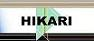| Home | Journals | Books | Paper submission | About us and our mission | News | Contact us |Site Search:

## Online Edition, Vol. 6, 2012, no. 57-60

Alaa M. F. AL. Jumaili, Xiao-Song Yang
A new types of upper and lower continuous multifunctions in topological spaces via e-open and e*-open sets
Int. Journal of Math. Analysis, Vol. 6, 2012, no. 57-60, 2803-2817.

K. Kannan, N. Nagaveni
On \cap{\beta}-generalized closed sets and open sets in topological spaces
Int. Journal of Math. Analysis, Vol. 6, 2012, no. 57-60, 2819-2828.

I. Ghanmi, R. Jebari, A. Boukricha
Homotopy perturbation method for solving elliptic partial differential equations
Int. Journal of Math. Analysis, Vol. 6, 2012, no. 57-60, 2829-2839.

I. Ghanmi, A. Boukricha
He's homotopy perturbation method for the reaction-type equation
Int. Journal of Math. Analysis, Vol. 6, 2012, no. 57-60, 2841-2856.

Yunxi Guo, Ying Wang
On existence of solutions for a periodic nonlinear dispersive wave equation
Int. Journal of Math. Analysis, Vol. 6, 2012, no. 57-60, 2857-2870.

A. Tajmouati
On topological divisor of zero and permanently singular elements in Jordan Banach algebras
Int. Journal of Math. Analysis, Vol. 6, 2012, no. 57-60, 2871-2884.

T. Jayakumar, K. Kanagarajan, S. Indrakumar
Numerical solution of Nth-order fuzzy differential equation by Runge-Kutta method of order five
Int. Journal of Math. Analysis, Vol. 6, 2012, no. 57-60, 2885-2896.

S. F. Hashemi
Some fixed point theorems for multi-valued contractions in quasi-metric spaces
Int. Journal of Math. Analysis, Vol. 6, 2012, no. 57-60, 2897-2902.

Guang Shi
Quasi–bases for modules over a commutative ring
Int. Journal of Math. Analysis, Vol. 6, 2012, no. 57-60, 2903-2910.

C. Lowongtrakool, N. Hiransakolwong
Noise filtering in unsupervised clustering using computation intelligence
Int. Journal of Math. Analysis, Vol. 6, 2012, no. 57-60, 2911-2920.

A. Gairola, M. C. Joshi
Coincidence points for mappings under generalized contraction
Int. Journal of Math. Analysis, Vol. 6, 2012, no. 57-60, 2921-2926.

F. Moukamba, D. Ampini, Y. Nkasa
Local controlability of a nonlinear heat equation with integral constraint
Int. Journal of Math. Analysis, Vol. 6, 2012, no. 57-60, 2927-2942.

M. R. Abdollahpour, A. Najati, Gwang Hui Kim
Hyers–Ulam stability of a differential equation of third order
Int. Journal of Math. Analysis, Vol. 6, 2012, no. 57-60, 2943-2948.

A. M. F. AL. Jumaili, Xiao-Song Yang
Fixed point theorems and \nabla^*-distance in partially ordered D*-metric spaces
Int. Journal of Math. Analysis, Vol. 6, 2012, no. 57-60, 2949-2955.

L. Karimi
Concave weighted shift operators
Int. Journal of Math. Analysis, Vol. 6, 2012, no. 57-60, 2957-2961.

H. Lakhbab, S. El Bernoussi
A hybrid method based on particle swarm optimization and nonmonotone spectral gradient method for unconstrained optimization problem
Int. Journal of Math. Analysis, Vol. 6, 2012, no. 57-60, 2963-2976.

H. Leiva, J. Matute, N. Merentes
On the Hammerstein-Volterra equation in the space of the absolutely continuous functions
Int. Journal of Math. Analysis, Vol. 6, 2012, no. 57-60, 2977-2999.